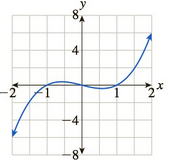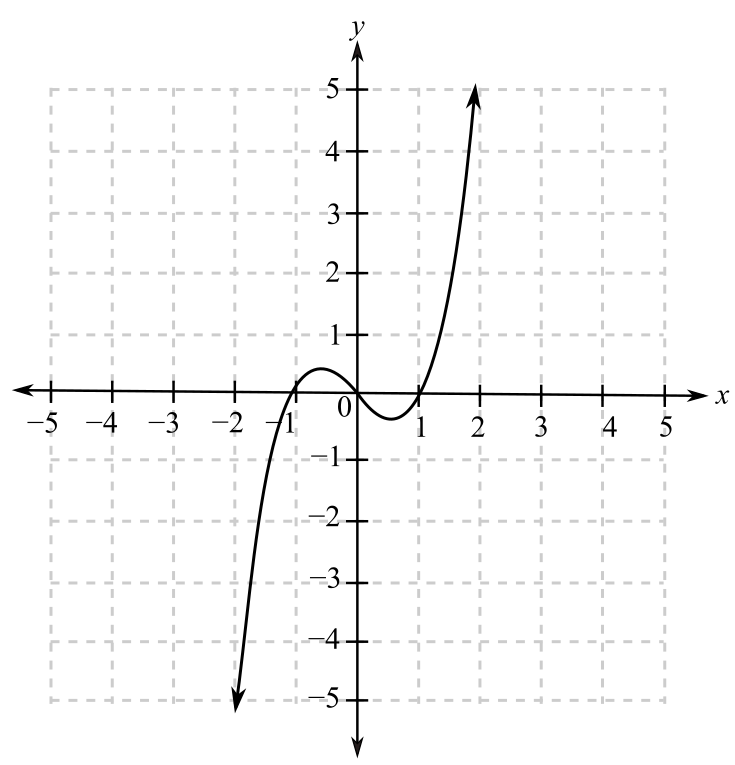Chapter 10.5, Problem 72EFinite Mathematics and Applied Cal...

7th Edition
Stefan Waner + 1 other
ISBN: 9781337274203

Solutions

Chapter
SectionFinite Mathematics and Applied Cal...

7th Edition
Stefan Waner + 1 other
ISBN: 9781337274203
Textbook Problem

In Exercises 69–72 the graph of the derivative of a function is given. For which x is the (original) function increasing? For which x is the (original) function decreasing? [HINT: See Quick Example 6.]To determine

The increasing and decreasing range of the function f from the graph of its derivative,Explanation

Given Information:

The provided graph is:

When the derivative of a function is positive in some interval then it is an increasing function in that interval and if the derivative is negative in some interval then it is a decreasing function in that interval.

From the graph, it can be observed that the derivative of the function is negative when x<1 and 0<x<1 whereas the derivative of the function is positive when 1<x<0 and x>1 which indicates that the function wo

Still sussing out bartleby?

Check out a sample textbook solution.

See a sample solution

The Solution to Your Study Problems

Bartleby provides explanations to thousands of textbook problems written by our experts, many with advanced degrees!

Get Started

Evaluate the indefinite integral. cos(1+5t)dt

Single Variable Calculus: Early Transcendentals, Volume I

In Exercises 31-34, find an equation of the line that passes through the points. 32. (2, 1) and (2, 5)

Applied Calculus for the Managerial, Life, and Social Sciences: A Brief Approach

True or False: is conservative.

Study Guide for Stewart's Multivariable Calculus, 8th